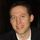cancel
Showing results for
Did you mean:Helper III

## Tricky IF DAX Formula

Hi Experts

I want to do the following as a either a if or Switch in a calculated column...

If (RTT[Actual_Weeks_Waiting] < 18, "Under 18",
If (RTT[Actual_Weeks_Waiting] >=18, "18+",
If (RTT[Actual_Weeks_Waiting] >=52, "52+",
If (RTT[Actual_Weeks_Waiting] >=72, "72+",
If (RTT[Actual_Weeks_Waiting] >=72, "78+",blank())))))

the above only returns back Under 18 and 18+
18+ should sum from 18 onwards to max value fine
52+ should some only values from 52 onwards to max and so on
1 ACCEPTED SOLUTIONSuper User

Try this calculated column:

``````CalculatedColumn =
SWITCH (
TRUE,
RTT[Actual_Weeks_Waiting] >= 78, "78+",
RTT[Actual_Weeks_Waiting] >= 72, "72+",
RTT[Actual_Weeks_Waiting] >= 52, "52+",
RTT[Actual_Weeks_Waiting] >= 18, "18+",
RTT[Actual_Weeks_Waiting] < 18, "Under 18",
BLANK ()
)``````

Proud to be a Super User!

7 REPLIES 7Memorable Member

Hi @Checkers111,

The problem statement that you have requires you to check for a range instead of checking if it is greater than a particular number. A number like 14 will result "Under 18" but since your second condition is >18, anything that is more than 18 (25, 53, 73, 79) will statisfy that condition and you will get "18+". The rest of the conditions in your code will never even be checked.

You should write the code for checking the ranges like this:

``````Category = SWITCH(TRUE,
'Table'[Values]<18, "Under 18",
'Table'[Values]>=18 && 'Table'[Values]<52, "18+",
'Table'[Values]>=52 && 'Table'[Values]<72, "52+",
'Table'[Values]>=72 && 'Table'[Values]<78, "72+")``````

Result:Did I answer your question? Mark this post as a solution if I did!Super User

Try this calculated column:

``````CalculatedColumn =
SWITCH (
TRUE,
RTT[Actual_Weeks_Waiting] >= 78, "78+",
RTT[Actual_Weeks_Waiting] >= 72, "72+",
RTT[Actual_Weeks_Waiting] >= 52, "52+",
RTT[Actual_Weeks_Waiting] >= 18, "18+",
RTT[Actual_Weeks_Waiting] < 18, "Under 18",
BLANK ()
)``````

Proud to be a Super User!Helper III

could you shed some light on the followingSuper User

I looked at your other post and noticed a missing close parenthesis at the end.

Proud to be a Super User!Helper III

Hi - even with that corrected its still not returning back the correct resultHelper III

Excellent sir worked.......Super User

Glad to hear it worked. By the way, you can remove the BLANK() argument since it will return BLANK if none of the conditions are met.

Proud to be a Super User!